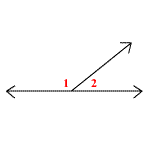# Unit 1 Assessment

The measure of an angle is 5 more than six times its supplement. a. How many angles are there? b. What are the angles' relationship? c. Find the measure of the largest angle.
 ﻿ Angle ﻿ Expression ﻿ Value (Degrees) x ﻿ 6(180-x)+5 ﻿ 155 ﻿Supplement ﻿ (180-x) (180-155) ﻿ 25
x=6(180-x)+5 x=1080-6x+5 x=1085-6x +6x +6x 7x=1085 7 7 x=155 Supplement= 180-155 Supplement=25Angle 1=155 degrees Angle 2=25 degrees
In the questions they ask how many angles there are,what is the relationship between the angles, and to find the largest angle. To answer, there is two angles and they share a supplementry relationship. This is because these two angles sum up to 180 degrees. To add on the largest angle is 155 degrees. I found this out by using the information above to come up with an equation, x=6(180-x)+5. I then solved it and found out the first angle was the largest angle. Finally I found the supplement by subtracting 155 from 180 to find that the second angle was 25 degrees.